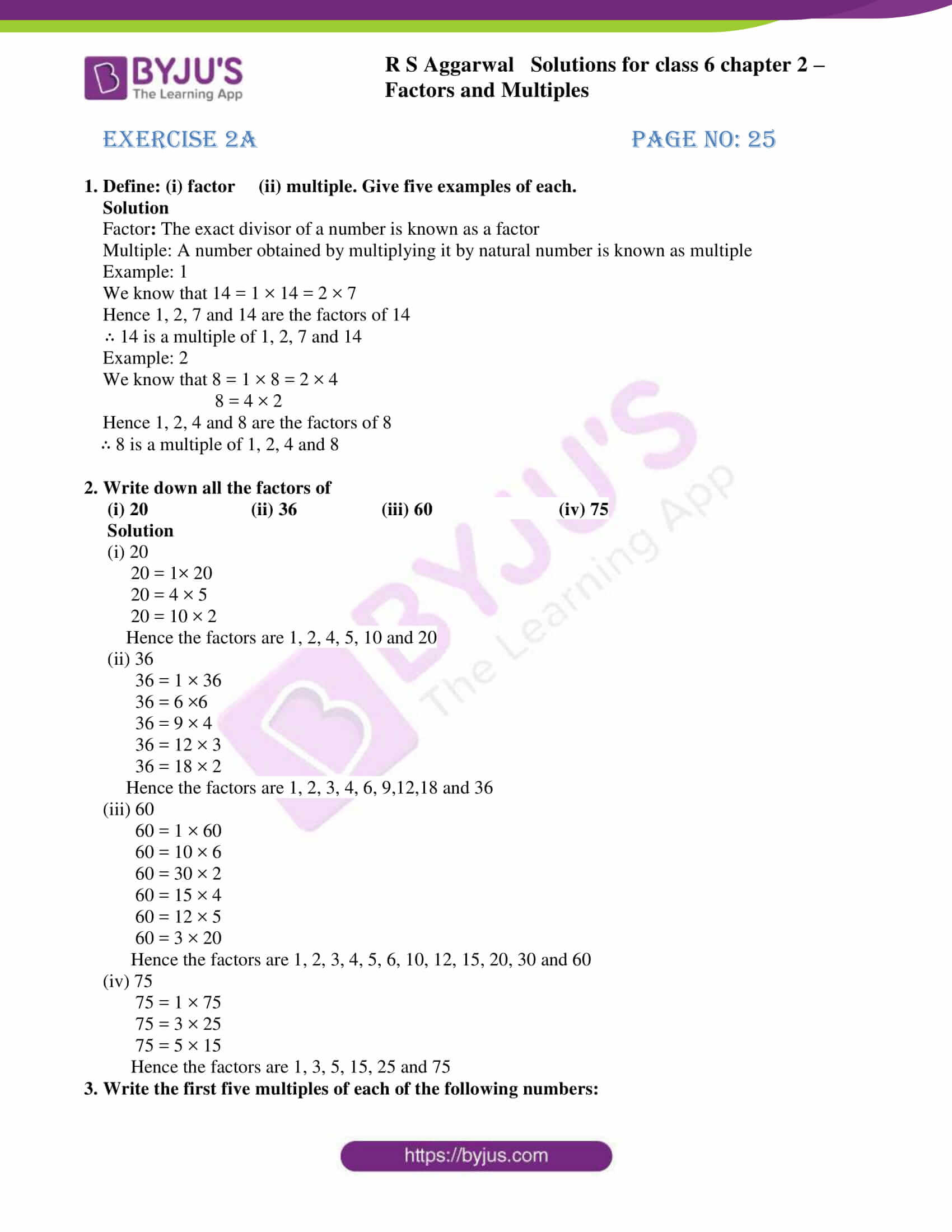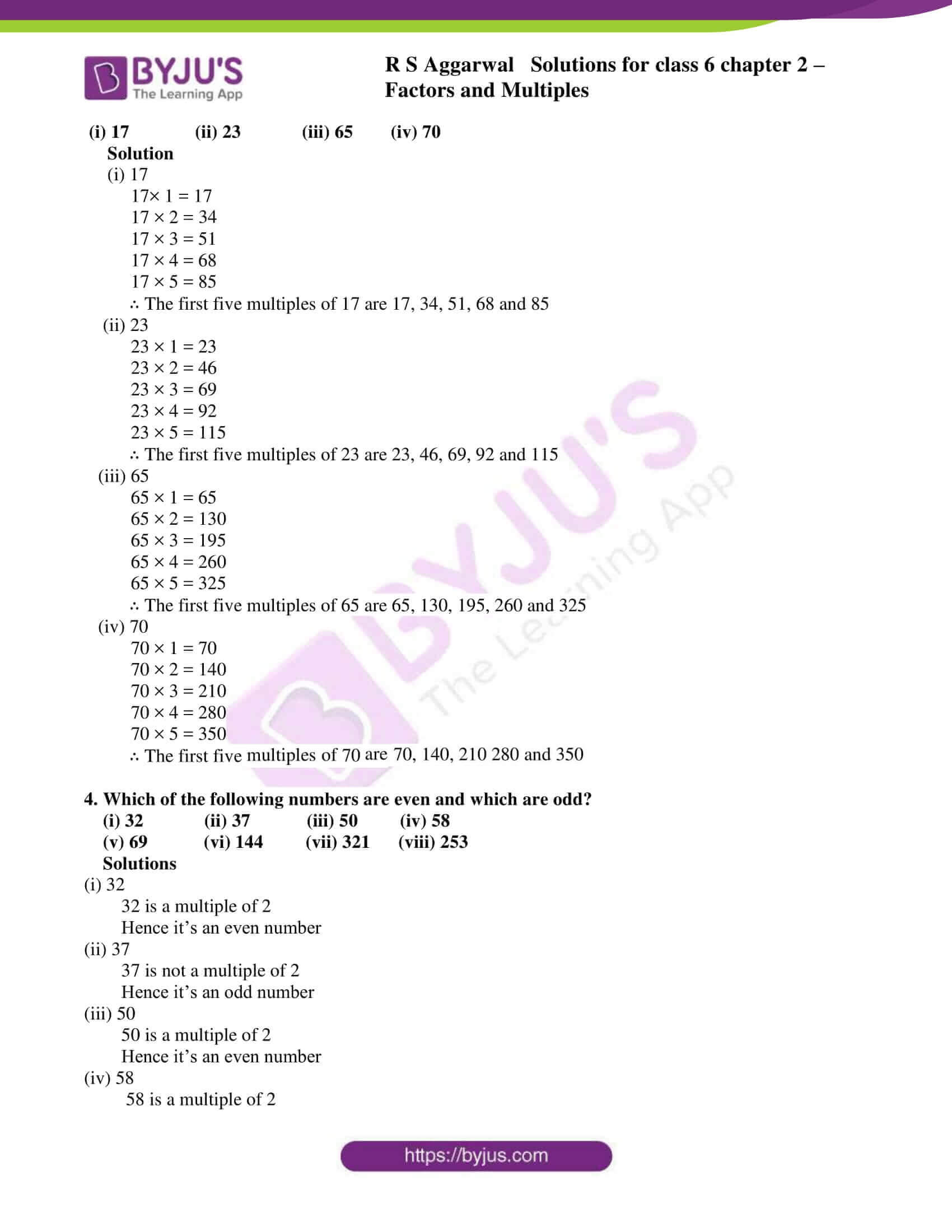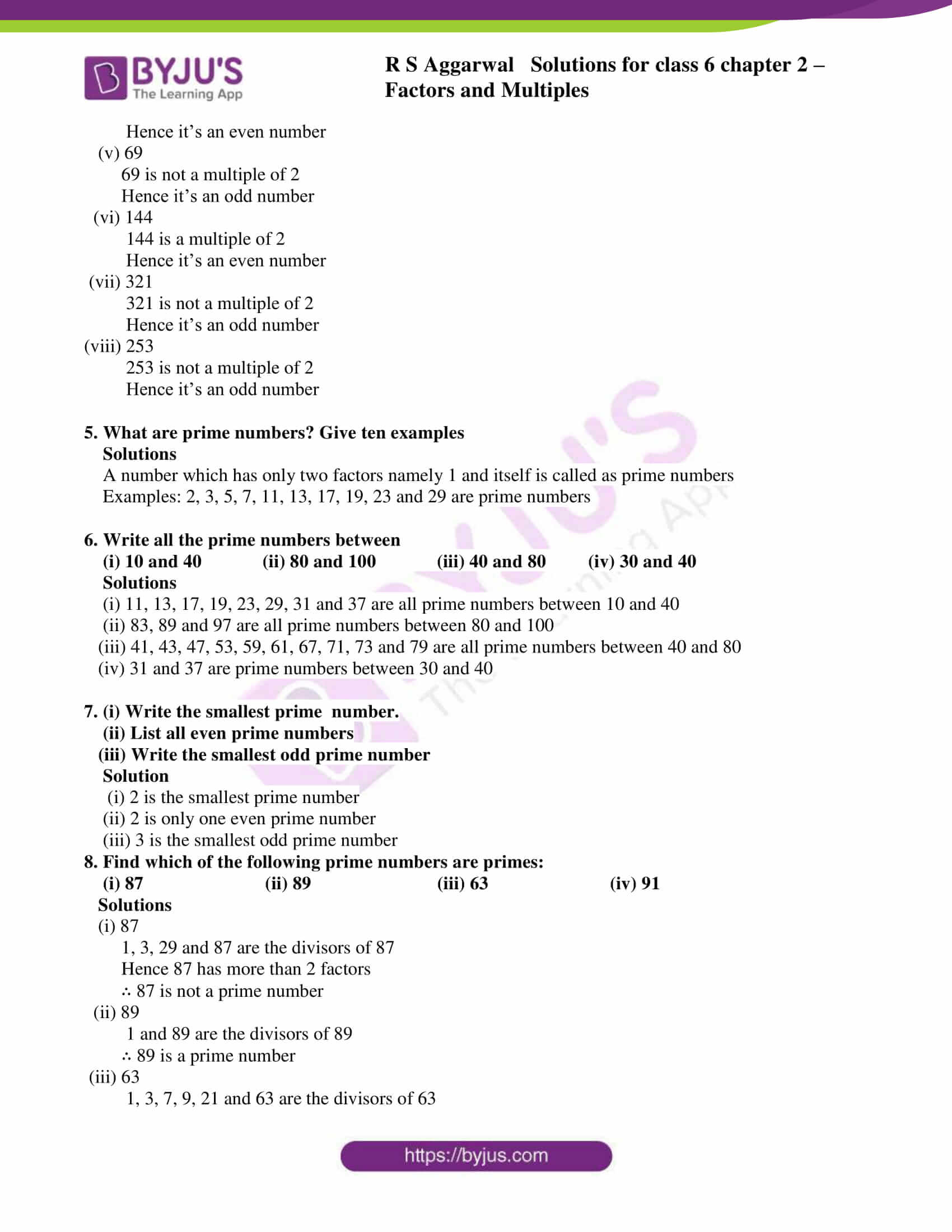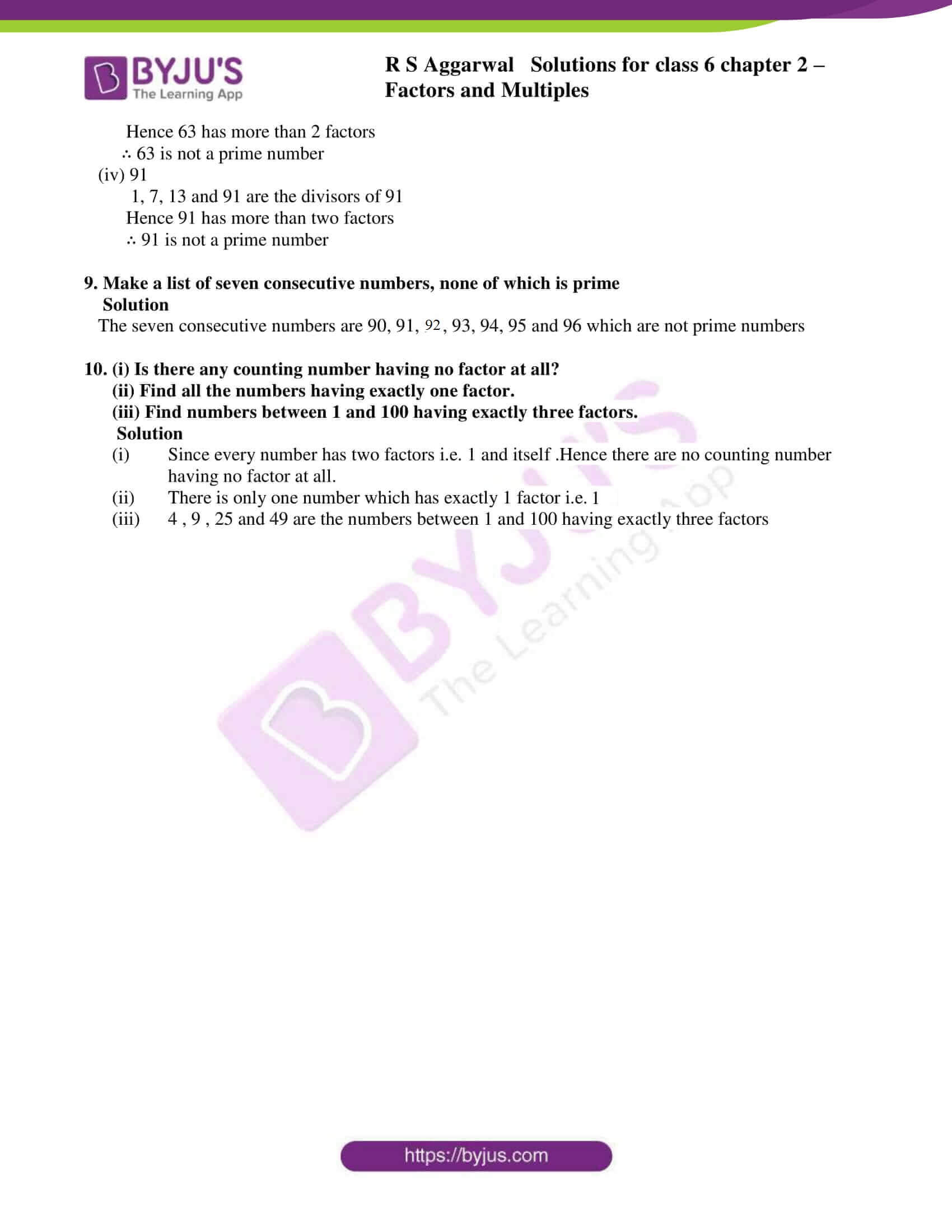# RS Aggarwal Solutions for Class 6 Maths Chapter 2 Factors and Multiples Exercise 2A

RS Aggarwal Solutions for Class 6 Maths, Chapter 2, Factors and Multiples Exercise 2A consists of the methods used in finding prime and composite numbers from 1 to 100. Students are advised to practice exercise wise problems using the solutions since the answers are well structured.

BYJU’S experts in Mathematics have prepared solutions with the primary aim of helping students score excellent marks in the examinations and improve conceptual knowledge in Mathematics.

## Download PDF of RS Aggarwal Solutions for Class 6 Maths Chapter 2 Factors and Multiples Exercise 2A### Access answers to Maths RS Aggarwal Solutions for Class 6 Chapter 2 Factors and Multiples Exercise 2A

1. Define: (i) factor (ii) multiple. Give five examples of each.

Solution

Factor: The exact divisor of a number is known as a factor

Multiple: A number obtained by multiplying it by natural number is known as multiple

Example: 1

We know that 14 = 1 × 14 = 2 × 7

Hence 1, 2, 7 and 14 are the factors of 14

∴ 14 is a multiple of 1, 2, 7 and 14

Example: 2

We know that 8 = 1 × 8 = 2 × 4

8 = 4 × 2

Hence 1, 2, 4 and 8 are the factors of 8

∴ 8 is a multiple of 1, 2, 4 and 8

2. Write down all the factors of

(i) 20 (ii) 36 (iii) 60 (iv) 75

Solution

(i) 20

20 = 1× 20

20 = 4 × 5

20 = 10 × 2

Hence the factors are 1, 2, 4, 5, 10 and 20

(ii) 36

36 = 1 × 36

36 = 6 ×6

36 = 9 × 4

36 = 12 × 3

36 = 18 × 2

Hence the factors are 1, 2, 3, 4, 6, 9,12,18 and 36

(iii) 60

60 = 1 × 60

60 = 10 × 6

60 = 30 × 2

60 = 15 × 4

60 = 12 × 5

60 = 3 × 20

Hence the factors are 1, 2, 3, 4, 5, 6, 10, 12, 15, 20, 30 and 60

(iv) 75

75 = 1 × 75

75 = 3 × 25

75 = 5 × 15

Hence the factors are 1, 3, 5, 15, 25 and 75

3. Write the first five multiples of each of the following numbers:

(i) 17 (ii) 23 (iii) 65 (iv) 70

Solution

(i) 17

17× 1 = 17

17 × 2 = 34

17 × 3 = 51

17 × 4 = 68

17 × 5 = 85

∴ The first five multiples of 17 are 17, 34, 51, 68 and 85

(ii) 23

23 × 1 = 23

23 × 2 = 46

23 × 3 = 69

23 × 4 = 92

23 × 5 = 115

∴ The first five multiples of 23 are 23, 46, 69, 92 and 115

(iii) 65

65 × 1 = 65

65 × 2 = 130

65 × 3 = 195

65 × 4 = 260

65 × 5 = 325

∴ The first five multiples of 65 are 65, 130, 195, 260 and 325

(iv) 70

70 × 1 = 70

70 × 2 = 140

70 × 3 = 210

70 × 4 = 280

70 × 5 = 350

∴ The first five multiples of 70 are 70, 140, 210 280 and 350

4. Which of the following numbers are even and which are odd?

(i) 32 (ii) 37 (iii) 50 (iv) 58

(v) 69 (vi) 144 (vii) 321 (viii) 253

Solutions

(i) 32

32 is a multiple of 2

Hence it’s an even number

(ii) 37

37 is not a multiple of 2

Hence it’s an odd number

(iii) 50

50 is a multiple of 2

Hence it’s an even number

(iv) 58

58 is a multiple of 2

Hence it’s an even number

(v) 69

69 is not a multiple of 2

Hence it’s an odd number

(vi) 144

144 is a multiple of 2

Hence it’s an even number

(vii) 321

321 is not a multiple of 2

Hence it’s an odd number

(viii) 253

253 is not a multiple of 2

Hence it’s an odd number

5. What are prime numbers? Give ten examples

Solutions

A number which has only two factors namely 1 and itself is called as prime numbers

Examples: 2, 3, 5, 7, 11, 13, 17, 19, 23 and 29 are prime numbers

6. Write all the prime numbers between

(i) 10 and 40 (ii) 80 and 100 (iii) 40 and 80 (iv) 30 and 40

Solutions

(i) 11, 13, 17, 19, 23, 29, 31 and 37 are all prime numbers between 10 and 40

(ii) 83, 89 and 97 are all prime numbers between 80 and 100

(iii) 41, 43, 47, 53, 59, 61, 67, 71, 73 and 79 are all prime numbers between 40 and 80

(iv) 31 and 37 are prime numbers between 30 and 40

7. (i) Write the smallest prime number.

(ii) List all even prime numbers

(iii) Write the smallest odd prime number

Solution

(i) 2 is the smallest prime number

(ii) 2 is only one even prime number

(iii) 3 is the smallest odd prime number

8. Find which of the following prime numbers are primes:

(i) 87 (ii) 89 (iii) 63 (iv) 91

Solutions

(i) 87

1, 3, 29 and 87 are the divisors of 87

Hence 87 has more than 2 factors

∴ 87 is not a prime number

(ii) 89

1 and 89 are the divisors of 89

∴ 89 is a prime number

(iii) 63

1, 3, 7, 9, 21 and 63 are the divisors of 63

Hence 63 has more than 2 factors

∴ 63 is not a prime number

(iv) 91

1, 7, 13 and 91 are the divisors of 91

Hence 91 has more than two factors

∴ 91 is not a prime number

9. Make a list of seven consecutive numbers, none of which is prime

Solution

The seven consecutive numbers are 90, 91, 92, 93, 94, 95 and 96 which are not prime numbers

10. (i) Is there any counting number having no factor at all?

(ii) Find all the numbers having exactly one factor.

(iii) Find numbers between 1 and 100 having exactly three factors.

Solution

(i) Since every number has two factors i.e. 1 and itself .Hence there are no counting number having no factor at all.

(ii) There is only one number which has exactly 1 factor i.e. 1

(iii) 4 , 9 , 25 and 49 are the numbers between 1 and 100 having exactly three factors

## RS Aggarwal Solutions for Class 6 Maths Chapter 2 Factors and Multiples Exercise 2A

RS Aggarwal Solutions for Class 6 Maths Chapter 2 Factors and Multiples Exercise 2A includes questions about the methods of finding prime and composite numbers from 1 to 100. Students can refer RS Aggarwal Solutions while solving exercise wise problems. Some of the topics included in other exercises are

• General properties of divisibility
• Prime factorization
• To find HCF
• Properties of HCF and LCM of given numbers

Practising exercise wise problems using RS Aggarwal Solutions helps the students to complete their homework without any obstacles. It also helps them to come out with flying colours in the exam.